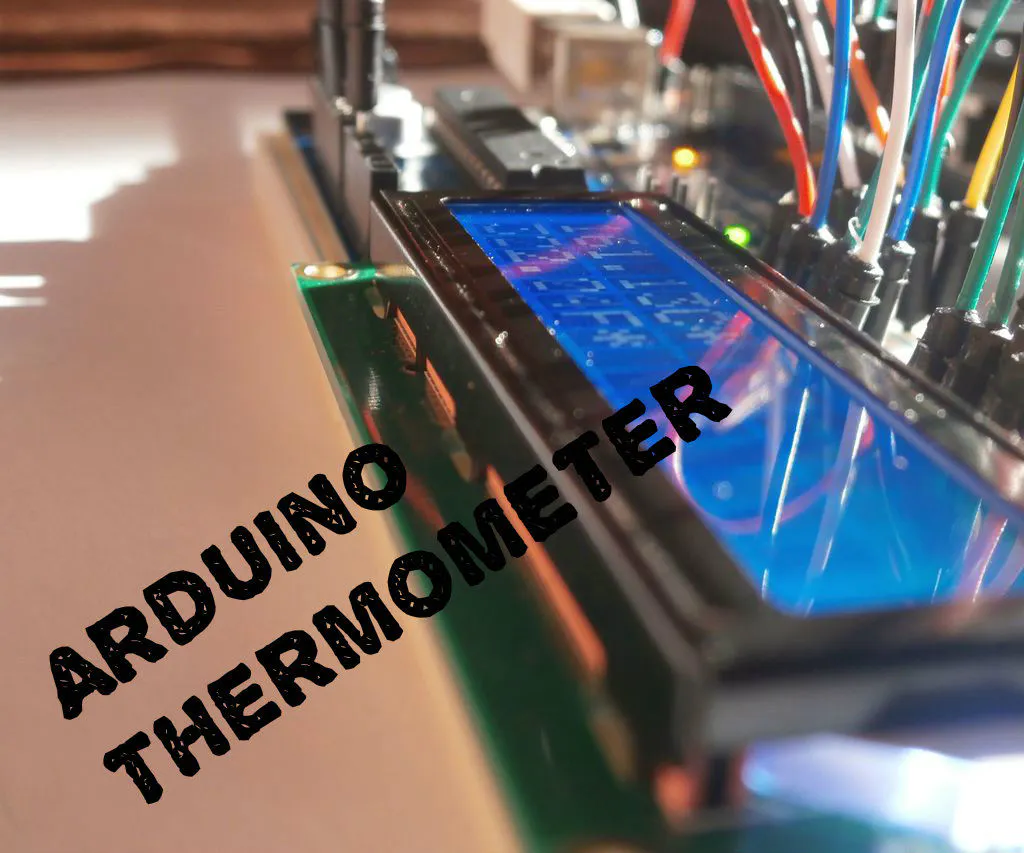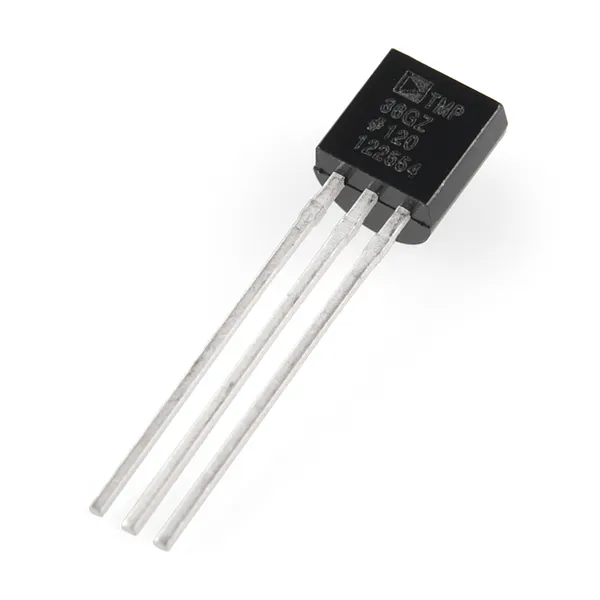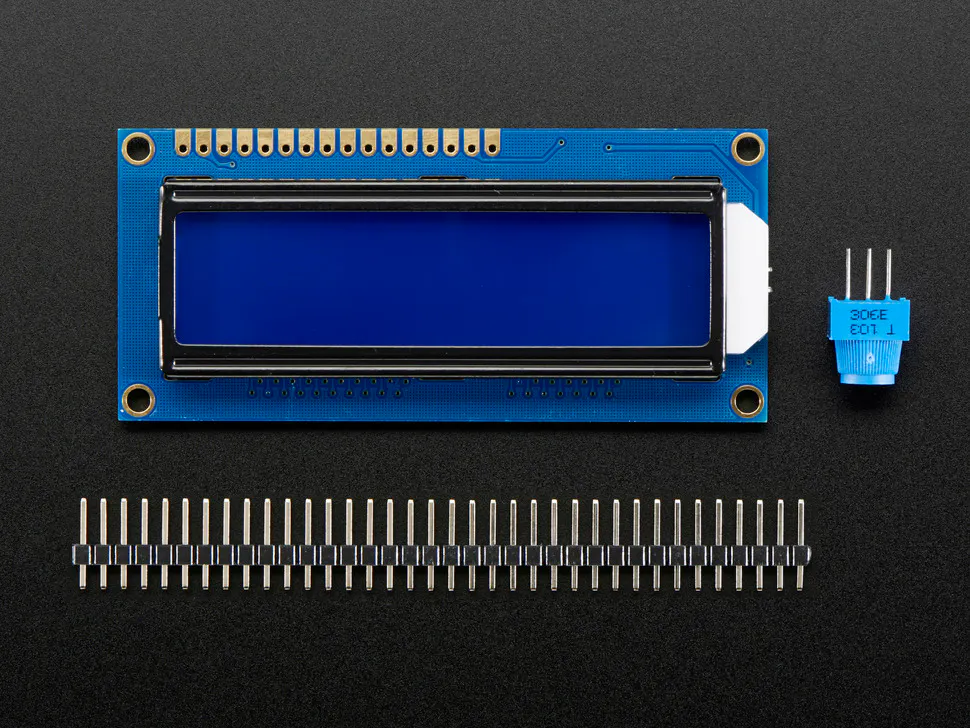# Making LCD Thermometer With Arduino And LM35/36

I'm going to show you how to make an LCD thermometer with an Arduino UNO and a LM35/36 analog temperature sensor.

BeginnerFull instructions provided9 minutes136,378## Things used in this project

### Hardware componentsArduino UNO
×1Temperature Sensor
×1Adafruit Standard LCD - 16x2 White on Blue
×1Jumper wires (generic)
×1×1

## Code

### Code snippet #1

Plain text
```<p>// include the library code #include

// initialize the library with the numbers of the interface pins
LiquidCrystal lcd(12, 11, 5, 4, 3, 2);
// initialize our variables
int sensorPin = 0;
int tempC, tempF;
void setup() {
// set up the LCD's number of columns and rows:
lcd.begin(16, 2);
}
void loop() {
tempC = get_temperature(sensorPin);
tempF = celsius_to_fahrenheit(tempC);
lcd.setCursor(0,0);
lcd.print(tempF); lcd.print(" "); lcd.print((char)223); lcd.print("F");
delay(200);
}
int get_temperature(int pin) {
// We need to tell the function which pin the sensor is hooked up to. We're using
// the variable pin for that above
// Read the value on that pin
// Calculate the temperature based on the reading and send that value back
float voltage = temperature * 5.0;
voltage = voltage / 1024.0;
return ((voltage - 0.5) * 100);
}
int celsius_to_fahrenheit(int temp) {
return (temp * 9 / 5) + 32;
}</p>
```

### Code snippet #2

Plain text
```#include <LiquidCrystal.h>

LiquidCrystal lcd(12, 11, 5, 4, 3, 2); //Digital pins to which you connect the LCD
const int inPin = 0;                   // A0 is where you connect the sensor
void setup()
{
lcd.begin(16,2);
}
void loop()
{
lcd.setCursor(0,1);
float millivolts = (value / 1024.0) * 5000;
float celsius = millivolts / 10;
lcd.clear();
lcd.setCursor(0,0);
lcd.print(celsius);
lcd.print("C");
lcd.setCursor(0,1);
lcd.print((celsius * 9)/5 + 32); //turning the celsius into fahrehait
lcd.print("F");
delay(1000);
}
```

## Credits

### Konstantin Dimitrov

11 projects • 173 followers